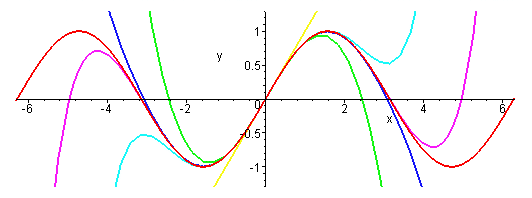## Friday, May 29, 2015

### Taylor's Series

In Appendix D of the 5th edition of Intermediate Physics for Medicine and Biology, Russ Hobbie and I review Taylor’s Series. Our Figures D.3 and D.4 show better and better approximations to the exponential function, ex, found by using more and more terms of its Taylor’s series. As we add terms, the approximation improves for small |x| and diverges more slowly for large |x|. Taking additional terms from the Taylor’s series approximates the exponential by higher and higher order polynomials. This is all interesting and useful, but the exponential looks similar to a polynomial, at least for positive x, so it is not too surprising that polynomials do a decent job approximating the exponential.

A more challenging function to fit with a Taylor’s Series would look nothing like a polynomial, which always grows to plus or minus infinity at large |x|. I wonder how the Taylor’s Series does approximating a bounded function; perhaps a function that oscillates back and forth a lot? The natural choice is the sine function.

The Taylor’s Series of sin(x) is

sin(x) = xx3/6 + x5/120 – x7/5040 + x9/362880 - …

The figure below shows the sine function and its various polynomial approximations.The sine function and its various polynomial approximations, from: http://www.peterstone.name/Maplepgs/images/Maclaurin_sine.gif
The red curve is the sine function itself. The simplest approximation is simply sin(x) = x, which gives the yellow straight line. It looks good for |x| less than one, but quickly diverges from sine at large |x|. The green curve is sin(x) = xx3/6. It rises to a maximum and then falls, much like sin(x), but  heads off to plus or minus infinity relatively quickly. The cyan curve is sin(x) = x – x3/6 + x5/120. It captures the first peak of the oscillation well, but then fails. The royal blue curve is sin(x) = xx3/6 + x5/120 – x7/5040. It is an excellent approximation of sin(x) out to x = π. The violet curve is sin(x) = xx3/6 + x5/120 – x7/5040 + x9/362880. It begins to capture the second oscillation, but then diverges. You can see the Taylor’s Series is working hard to represent the sine function, but it is not easy.

Appendix D in IPMB gives a table of values of the exponential and its different Taylor’s Series approximations. Below I create a similar table for the sine. Because the sine and all its series approximations are odd functions, I only consider positive values of x.A table of values for sin(x) and its various polynomial approximations.

One final thought. Russ and I title Appendix D as “Taylor’s Series” with an apostrophe s. Should we write “Taylor Series” instead, without the apostrophe s? Wikipedia just calls it the “Taylor Series.” I’ve seen it both ways, and I don’t know which is correct. Any opinions?

#### 1 comment:

1.I vote for "Taylor Series." In Bill Bialek's recent biophysics book there's a nice footnote on Reynolds number, Bessel functions, and other apostrophe-less things: https://books.google.com/books?id=5In_FKA2rmUC&lpg=PA154&dq=bialek%20reynolds%20number&pg=PA154#v=onepage&q&f=false